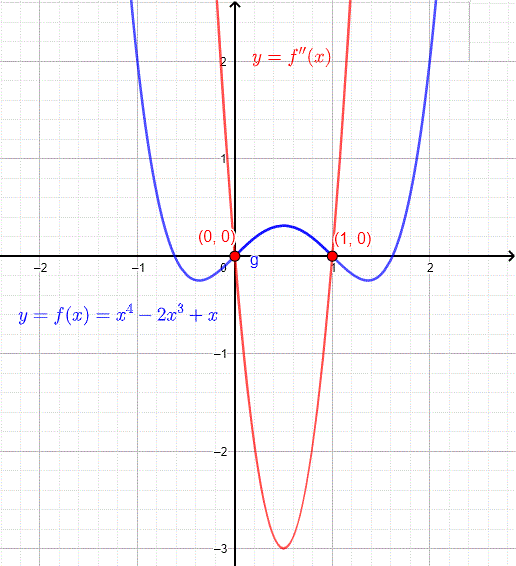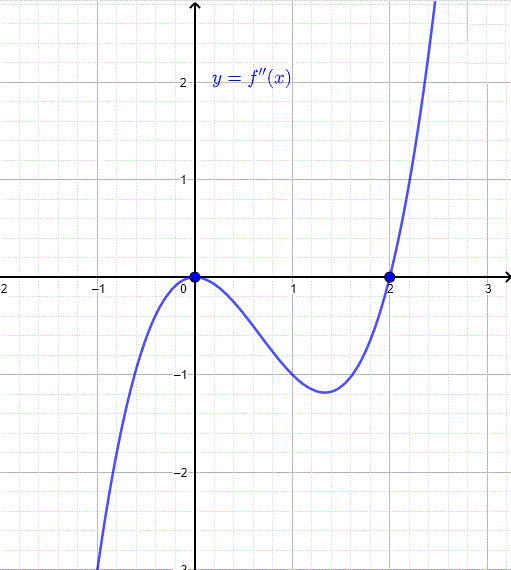# Concavity and Point of Inflection of Graphs

The definition of the concavity of a graph is introduced along with inflection points. Examples, with detailed solutions, are used to clarify the concept of concavity.

## Example 1: Concavity Up

Let us consider the graph below. Note that the slope of the tangent line (first derivative) increases. The graph in the figure below is called concave up.Figure 1

## Example 2: Concavity Down

The slope of the tangent line (first derivative) decreases in the graph below. We call the graph below concave down.Figure 2

## Definition of Concavity

Let f ' be the first derivative of function f that is differentiable on a given interval I, the graph of f is
(i) concave up on the interval I, if f ' is increasing on I
, or
(ii) concave down on the interval I, if f ' is decreasing on I.

The sign of the second derivative informs us when is f ' increasing or decreasing.

## Theorem

Let f '' be the second derivative of function f on a given interval I, the graph of f is
(i) concave up on I if f ''(x) > 0 on the interval I.
(ii) concave down on I if f ''(x) < 0 on the interval I.

## Definition of Point of Inflection

A point P on the graph of y = f(x) is a point of inflection if f is continuous at P and the concavity of the graph changes at P. In view of the above theorem, there is a point of inflection whenever the second derivative changes sign.

## Example 3

Determine the values of the leading coefficient a for which the graph of function f(x) = a x 2 + b x + c is concave up or down.

## Solution to Example 3

We first find the first and second derivatives of function f.
f '(x) = 2 a x + b
f ''(x) = 2 a
We now study the sign of f ''(x) which is equal to 2 a. If a is positive, f ''(x) is positive in the interval (-∞ , + ∞). According to the theorem above, the graph of f will be concave up for positive values of a.
If a is negative, the graph of f will be concave down on the interval (-∞ , + ∞) since f ''(x) = 2 a is negative.

The graphs of two quadratic functions are shown below: y = 2 x^2 - 2 x - 1 whose graph is convcave up because its leading coefficient (a = 2) is positive and y = - x^2 + 3 x + 1 whose graph is convcave down because its leading coefficient (a = -1) is negative.## Example 4

a) Find the intervals on which the graph of f(x) = x 4 - 2x 3 + x is concave up, concave down and the point(s) of inflection if any.
b) Use a graphing calculator to graph f and confirm your answers to part a).

## Solution to Example 4

Let us find the first two derivatives of function f.
a)
f '(x) = 4 x
3 - 6 2 + 1
f ''(x) = 12
2 - 12 x
Find the zeros f ''(x).
12
2 - 12 x = 0
12 x (x - 1) = 0
Two zeros
x = 0 and x = 1
Study sign of f ''
The two zeros split the set of real numbers into three intervals. Select a value for x in each of the three intervals and find the sign of f''We now use the table of sign and the theorem above to conclude that
in the interval (-∞ , 0); f '' is positive and therefore the graph of f is concave up
in the interval (0 , 1); f '' is negative and therefore the graph of f is concave down
in the interval (1 , +∞); f '' is positive and therefore the graph of f is concave up
The second derivative f '' changes sign at x = 0 and x = 1 and therefore the graph of f has two inflection point: (0 , f(0)) and (1 , f(1))

b)
The graph of f (blue) and f '' (red) are shown below. It can easily be seen that whenever f '' is negative (its graph is below the x-axis), the graph of f is concave down and whenever f '' is positive (its graph is above the x-axis) the graph of f is concave up.
Point (0,0) is a point of inflection where the concavity changes from up to down as x increases (from left to right) and point(1,0) is also a point of inflection where the concavity changes from down to up as x increases (from left to right).## Example 5

The graph of the second derivative f '' of function f is shown below. Find the intervals where f is concave up, concave down and the point(s) of inflection if any.## Solution to Example 5

According to the graph of f '', the sign of f '' is given over the following intervals
a)
On the interval (-∞ , 2), the graph of f '' is below the x axis and therefore f '' is negative, hence f is concave down on the interval (-∞ , 2)
On the interval (2 , +∞), the graph of f '' is above the x axis and therefore f '' is positive, hence f is concave up on the interval (2 , +∞)
At x = 2, the sign of f '' changes and therefore x = 2 is a point of inflection.

## Example 6

The graph of the first derivative f ' of function f is shown below. Find the intervals where the graph of f is concave up, concave down and the point(s) of inflection if any.## Solution to Example 6

We use the graph of the first derivative f ' to find the sign of the second derivative and deduce the concavity of the graph of f
a)
On the interval (-∞ , -2), f ' decreases and therefore f '' is negative; the graph of f is concave down
On the interval (-2 , -1), f ' increases and therefore f '' is positive; the graph of f is concave up
On the interval (-1 , 1), f ' decreases and therefore f '' is negative; ; the graph of f is concave down
On the interval (1 , ∞), f ' increases and therefore f '' is positive; ; the graph of f is concave up
The concavity of the graph of f changes at x = -2, x = -1 and x = 1 and therefore these are all point of inflection.